# Time Dependent Acceleration

If a time dependent acceleration can be expressed as a polynomial in time, then the velocity and position can be obtained, provided the appropriate initial conditions are known. Allowing the acceleration to have terms up to the second power of time leads to the following motion equations for one dimensional motion.Variable acceleration Polynomial integrals Polynomial derivatives Calculation
 Example problem
Index

Motion concepts

Velocity and acceleration

 HyperPhysics***** Mechanics R Nave
Go Back

# Motion Calculation, Variable Acceleration

For a variable acceleration which can be expressed as a polynomial in time, the position and velocity can be calculated provided their initial values are known. .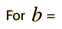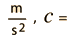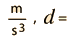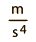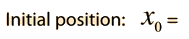m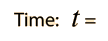seconds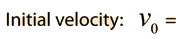m/sm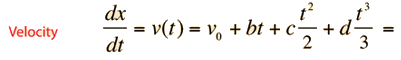m/sm/s2

Note that even in this one-dimensional case for non-constant acceleration, the average velocity is not equal to the average of the initial and final velocities. The average velocity must be calculated from:m/s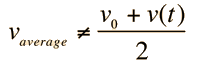m/s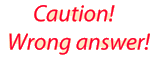The average velocity expression from the constant acceleration equations works only for constant acceleration where the graph of velocity as a function of time is a straight line, the average being the midpoint of that line over the chosen time interval. If you make c and d in the above expressions equal to zero, dropping back to constant acceleration motion, then the two expressions for average acceleration will give the same answer.
Index

Motion concepts

Velocity and acceleration

 HyperPhysics***** Mechanics R Nave
Go Back